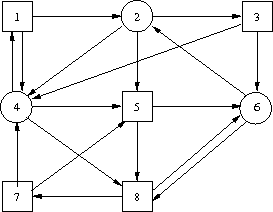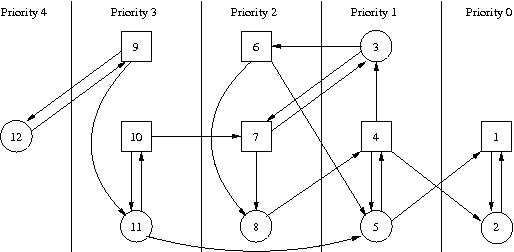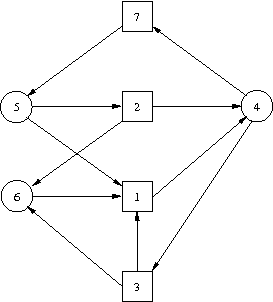# LAT Homework

Consider the automata construction from proof of the Myhill-Nerode theorem for a language L. Prove

• (a) the constructed automaton A is the minimal automaton (in the number of states) that recognizes L;
• (b) any minimal automaton recognizing L is isomorphic to A.
1. Given a reachability game (G,F), give an algorithm that computes the 0-Attractor(F) set in time O(|E|).
2. Consider the game graph shown in below and the following winning conditions: a) Occ(ρ) ∩ {1} ≠ ∅ and b) Occ(ρ) ⊆ {1,2,3,4,5,6} and c) Inf(ρ) ∩ {4,5} ≠ ∅. Compute the winning regions and corresponding winning strategies showing the intermediate steps (i.e., the Attractor and Recurrence sets) of the computation.3. Given a game graph G=(S,S0,T) and a set F ⊆ S. Let W0 and W_1 be the winning regions of Player 0 and Player 1, respectively, in the Buchi game (G,F). Prove or disprove:
1. The winning set of Player 0 in the safety game for (G,W0) is W0,
2. If f0 is a winning strategy for Player 0 in the safety game for (G,W0), then f0 is also a winning strategy for Player 0 in the Buchi game for (G,F),
3. the winning set of Player 1 in the guaranty game for (G,W_1) is W_1, and
4. if f_1 is a winning strategy for Player 1 in the guaranty game for (G,W0), then f_1 is a winning strategy in the Buchi game (G,F).
1. Consider the game graph shown below. Let the winning condition for Player 0 be Occ(ρ)={1,2,3,4,5,6,7}
1. Find the winning region for Player 0 and describe a winning strategy
2. Show that there is no positional winning strategy for Player 0 in this game.2. Compute the winning regions and the corresponding positional winning strategies for Player 0 and 1 in the weak-parity game shown below.3. A winning strategy is called “uniform” if it is a winning strategy from every winning state in the game. Let (G,p) be a weak parity game and let W0 be the winning region of Player 0. For all s ∈ W0 let f_s be a positional winning strategy from s for Player 0. Construct a uniform winning strategy f from the strategies f_s meaning that for every s ∈ W0 there is a t ∈ W0, s.t. f(s) = f_t(s).
1. Consider the game graph below and the Muller condition F={{2,4,5,7},{1,2,3,4,5,6,7}}. Find an automaton winning strategy for Player 0 in the Muller game with as few states as possible and show that any other automaton strategy with less states is not winning for Player 0.2. Consider the game shown on page 6 of slide10.pdf. We propose the following “latest appearance queue” (LAQ) strategy for Player 0, initializing the queue with s1s2s3s4,
1. add the current state at the front of the LAQ and delete the last state
2. move to the state ti whose number i is the number of different states in the current LAQ,
e.g., for the sequence of states s1, s3, s3, s4, s1,… we obtain the following sequence of LAQs: s1s2s3s4, s1s1s2s3, s3s1s1s2, s3s3s1s1, s4s3s3s1,s1s4s3s3,…
Decide wheater the new LAQ strategy is a winning strategy for Player 0. Prove this or give a counter-example.
3. Let A=(S,s0,T,p) be a parity automaton with p:S → {1,..,k}. Construct a Streett automaton B=(S,s0,T,F) equivalent to A that has the same transition structure as A. (i.e., find a suitable set of Streett pairs (F_i,E_i))

- Exam-like problems on automata and Presburger Arithmetic homework.pdf